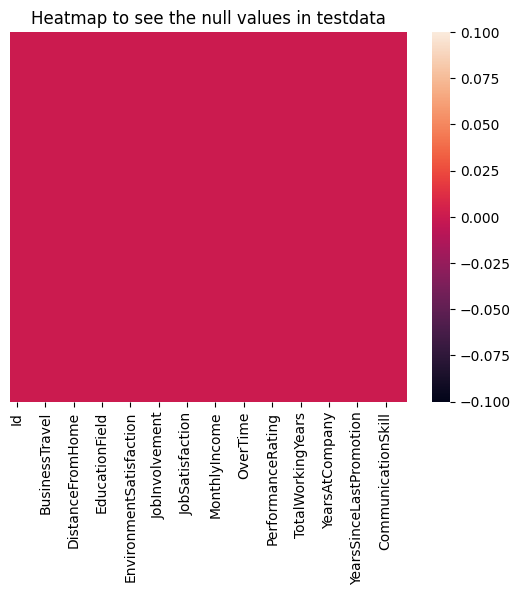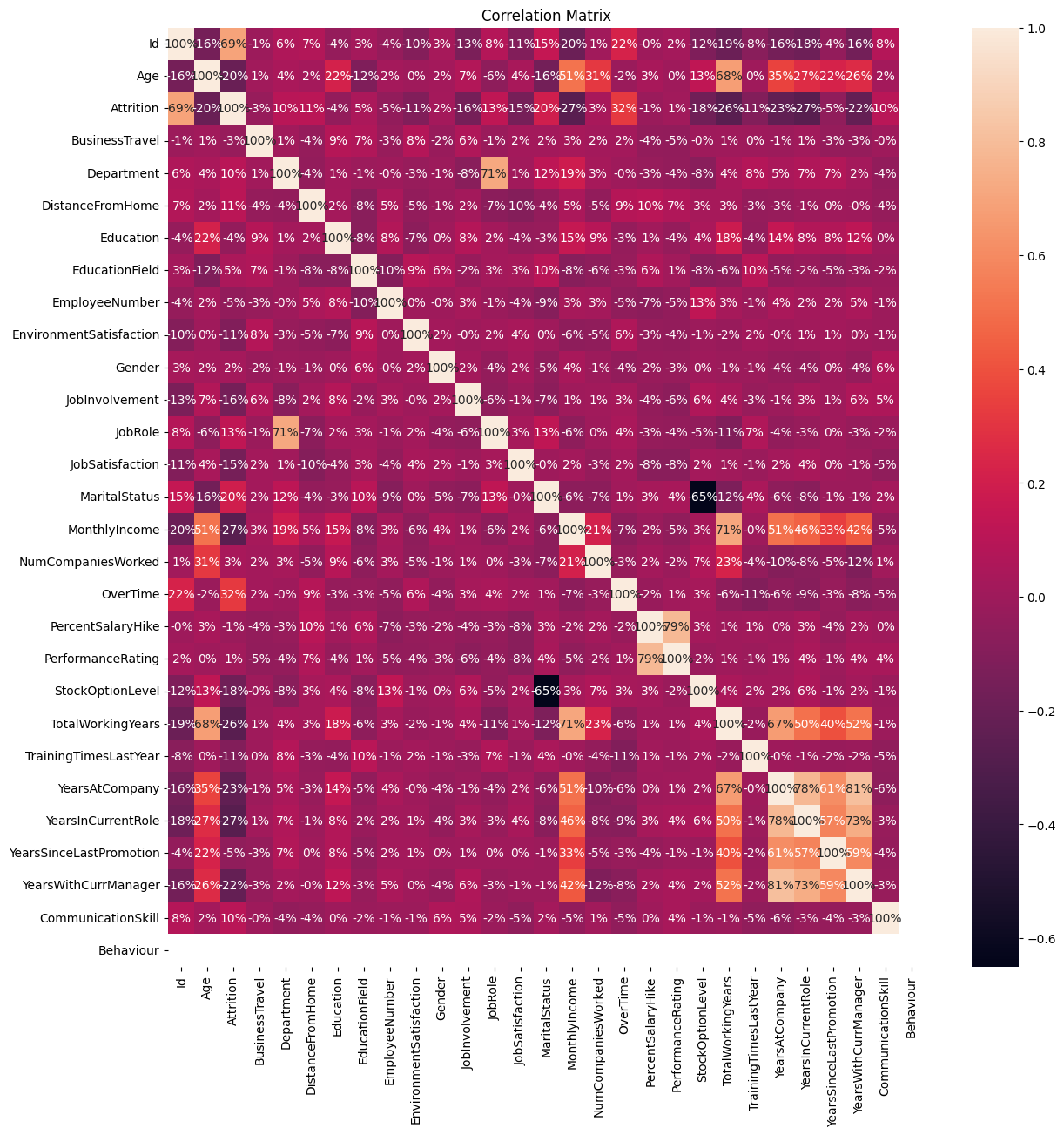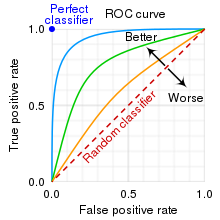# Prediction of Employee Attrition using Machine Learning

## Prediction of Employee Attrition using Machine LearningLets learn how machine learning can be used to predict the employee attrition. The goal of the blog is to give you complete walkthrough of all the steps involved in solving a problem i.e. prediction of employee attrition using machine learning.

### Table of Content:

1. What is Employee Attrition?
2. Data Collection
3. Exploratory Data Analysis
4. Feature Engineering and Feature Selection
5. Performance Metrics
6. Model Training using Logistic regression Model
7. Model Training using Decision Tree Model
8. Testing on Test data
9. Model Deployment
10. Summary of the Article
11. References

### What is Employee Attrition?

Employee attrition is defined as employees leaving their organizations for unpredictable or uncontrollable reasons. Many terms make up attrition, the most common being termination, resignation, planned or voluntary retirement, structural changes, long-term illness, and layoffs. In this blog, we will predict the attrition of employees using machine learning algorithms and deploy the model in the cloud.

### Data Collection

The data is available in this competition on Kaggle.Kaggle is an online community of data scientists and machine learning engineers. Kaggle allows users to find datasets they want to use in building AI models, publish datasets, work with other data scientists and machine learning engineers, and enter competitions to solve data science challenges. IBM provided this dataset. The dataset has train.csv file and test.csv file. The meaning of each feature is also explained. Let’s import all the necessary libraries and see the records available in the csv file using python.

``````import numpy as np
import pandas as pd
import matplotlib.pyplot as plt
import seaborn as sns
%matplotlib inline

from sklearn.preprocessing import LabelEncoder
from sklearn.model_selection import train_test_split
from sklearn.ensemble import RandomForestClassifier
from sklearn.linear_model import LogisticRegression
from sklearn.tree import DecisionTreeClassifier
from sklearn.metrics import accuracy_score
from sklearn.metrics import accuracy_score,roc_auc_score,roc_curve

import warnings
warnings.filterwarnings('ignore')``````

The below code will mount google drive into colab as the csv files are in google drive.

``````from google.colab import drive
drive.mount('/content/gdrive/', force_remount=True)``````
```Mounted at /content/gdrive/ ```

Then we will load the train data and test data and see the first 5 rows of these data.

``````traindf = pd.read_csv('/content/gdrive/MyDrive/Jovian/employee data/train.csv')
``traindf.head()``
``testdf.head()``
``traindf.shape``
``(1628, 29)``
``testdf.shape``
``(470, 28)``

### Exploratory Data Analysis

There are 1628 training data and 470 testing data. We can see that most of the features are numerical and some features are categorical in nature like BusinessTravel, Department, EducationField, Gender. We can also see that training data has a feature attrition and it is filled with 0 and 1, where 0 means the employee do not leave the company and 1 means the employee leave the company.

Next, we will check, if there are any missing values present in the dataset using isna function and using heatmap.

``traindf.isna().sum()``
``````Id                         0
Age                        0
Attrition                  0
Department                 0
DistanceFromHome           0
Education                  0
EducationField             0
EmployeeNumber             0
EnvironmentSatisfaction    0
Gender                     0
JobInvolvement             0
JobRole                    0
JobSatisfaction            0
MaritalStatus              0
MonthlyIncome              0
NumCompaniesWorked         0
OverTime                   0
PercentSalaryHike          0
PerformanceRating          0
StockOptionLevel           0
TotalWorkingYears          0
TrainingTimesLastYear      0
YearsAtCompany             0
YearsInCurrentRole         0
YearsWithCurrManager       0
CommunicationSkill         0
Behaviour                  0
dtype: int64``````
``testdf.isna().sum()``
``````Id                         0
Age                        0
Department                 0
DistanceFromHome           0
Education                  0
EducationField             0
EmployeeNumber             0
EnvironmentSatisfaction    0
Gender                     0
JobInvolvement             0
JobRole                    0
JobSatisfaction            0
MaritalStatus              0
MonthlyIncome              0
NumCompaniesWorked         0
OverTime                   0
PercentSalaryHike          0
PerformanceRating          0
StockOptionLevel           0
TotalWorkingYears          0
TrainingTimesLastYear      0
YearsAtCompany             0
YearsInCurrentRole         0
YearsWithCurrManager       0
CommunicationSkill         0
Behaviour                  0
dtype: int64``````
``````plt.title('Heatmap to see the null values in testdata')
sns.heatmap(testdf.isnull(), yticklabels = False)``````
``<Axes: title={'center': 'Heatmap to see the null values in testdata'}>``We can see that train data and test data have no null values. Next, we are converting the categorical data into numerical data using label encoder.Computer only understands 0 and 1. It cannot understand the text data directly.So, we are using label encoder to encode the text data into 0 and 1 form.Let’s take an example to understand. The Gender feature has only two values i.e. male and female. So label encoder will replace female with 0 and male with 1.

``````nominal_catg_col = list(traindf.select_dtypes(['object']).columns)
print(nominal_catg_col)``````
```['BusinessTravel', 'Department', 'EducationField', 'Gender', 'JobRole', 'MaritalStatus', 'OverTime'] ```

The above code shows all the columns where we need to do label encoding.

``````for column in traindf.columns:
if traindf[column].dtype == np.number:
continue
traindf[column] = LabelEncoder().fit_transform(traindf[column]) ``````
``````for column in testdf.columns:
if testdf[column].dtype == np.number:
continue
testdf[column] = LabelEncoder().fit_transform(testdf[column]) ``````

### Feature Engineering and Feature Selection

We are checking the correlation of the target variable with other features and plotting this using the seaborn. A correlation matrix is simply a table which displays the correlation coefficients for all the possible pairs of features. Correlation will check how a given features is affecting the attrition features. 100% means very strong correlation and -ve % correlation means weekly correlated. We can see that feature like Id, EmployeeNumber, Behaviour has -ve% correlation value. So, we can say that these feature will be less important while choosing the attrition and we will drop these features from the train data and test data.

``````plt.figure(figsize=(14,14))
plt.title('Correlation Matrix')
sns.heatmap(traindf.corr(), annot=True, fmt='.0%')``````
``<Axes: title={'center': 'Correlation Matrix'}>````````cols = ['Id', 'EmployeeNumber', 'Behaviour']

traindf.drop(traindf[cols], axis = 1, inplace=True)
testdf.drop(testdf[cols], axis = 1, inplace = True)``````

### Performance Metrics

Measuring the performance of a machine learning model is very crucial. The AUC (Area under the curve) - ROC (Receiver Operating Characteristics) curve is used as a performance metric in classification problems. The classification problem is the problem where we need to classify the data as positive or negative e.g. here we are classifying the whether the employee will leave the company or not based on the available features. ROC is a probability curve and AUC represents the degree or measure of separability. AUC score lies between 0 to 1. If AUC score is 1 then the model perfectly classifies all data points. If AUC score is 0 then the model is classifying the positive data points as negative and negative data points as positive. The figure shows the ROC curve and the actual area that comes under the ROC curve is AUC.If you want to know more about AUC-ROC curve then read this blog

### Model Training using Logistic Regression Model

We need to split the training data into train and test data using Scikit-Learn. The dataset will be divided into training dataset and validation dataset. Training dataset is used to fit the model and validation dataset is used to train the model. The objective is to estimate the model performance on completely new data.

``````X = traindf.drop('Attrition', axis=1)
Y = traindf['Attrition']``````
``X_train, X_test, y_train, y_test = train_test_split(X,Y, test_size = 0.30)``

Logistic Regression is a statistical model used to model a classification problem by analyzing the relationship between the target variable and independent variable. The model estimates the probability of the outcome based on the values of the predictor variables. If you want to know more about Logistic regression then read this blog . We will create an object of ml algorithm then fit the model with the training data (X_train), predict the output in validation data (X_test), calculate the accuracy, draw ROC curve and calculate AUC score.

``````model_1 = LogisticRegression(C = 1, max_iter=1000)    #Create an object of ml algorithm

model_1.fit(X_train, y_train)            #train our model
preds_1 = model_1.predict(X_test)        #testing our model

accuracy_1 = accuracy_score(y_test, preds_1)  #calculate accuracy
print("Accuracy of the model is:", accuracy_1*100)

roc_auc_1 = roc_auc_score(y_test, model_1.predict(X_test))

fpr_1, tpr_1, thresholds_1 = roc_curve(y_test,model_1.predict_proba(X_test)[:,1])
plt.figure()

plt.plot(fpr_1, tpr_1, label='Logistic Regression (area = %0.2f)' % roc_auc_1)
plt.plot([0, 1], [0, 1],'r--')
plt.xlim([0.0, 1.0])
plt.ylim([0.0, 1.05])
plt.xlabel('False Positive Rate')
plt.ylabel('True Positive Rate')
```Accuracy of the model is: 78.11860940695297 ```